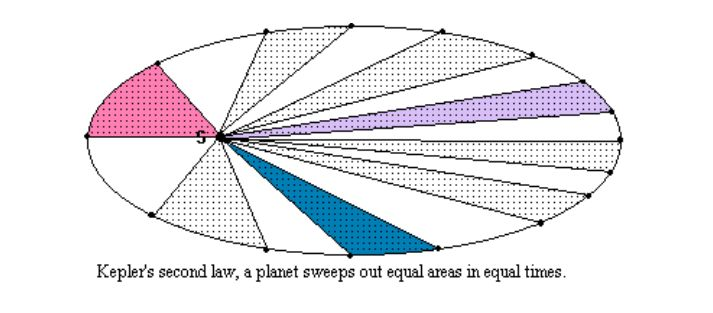2018-05-05 乔治·斯坦科夫 启动EVENT宇宙法则

乔治·斯坦科夫S U = c 2 /G = [1d- 空间 ]-

ε = 0.5(r max – r min )/a = 0 SP(A) 1.开普勒第二定律，行星扫描出在相同时间的相同区域。

M mol = m p (n pr f c,pr + n n f c,n +n e f c,e )nN A ,

ε = Δr/2a = (r max – r min )/2a.

ε = Δr/2a = f o /Δ f max = SP(A)

E 斥力 : E 吸引力 = ρ 斥力 :

ρ 吸引力 =v e(max) : v a(max) = 常数 = 1

注释：

1. 它可以证明香农的信息定义是一个基本术语的迭代。

2. PA Tipler 著，《物理学教科书》， 531 页（旧版）

3. 如见， K. Lancaster 著，《现代微观经济学导论》，芝加哥兰德麦克纳利学院出版社， 1974 年，第 12 页。

编译 | 马克兔文【相关阅读】